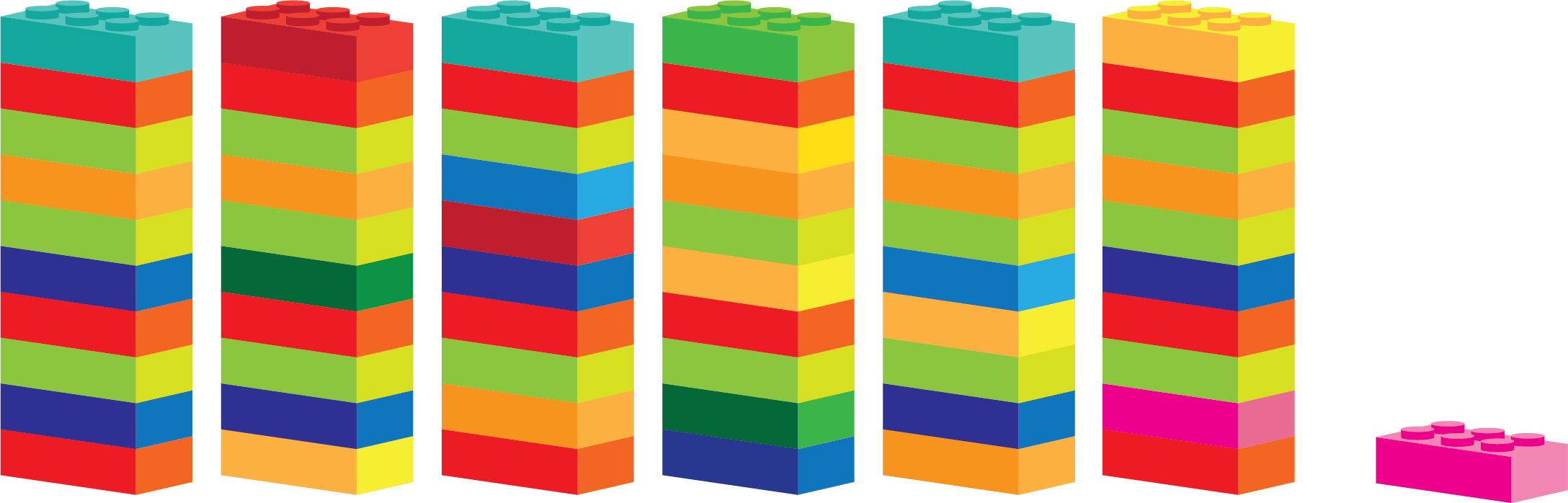# Learning About the Clock (Hours)

When you do things like going to a birthday party or going to school, you’re usually there for a long time, which is a lot of minutes. It can be difficult to describe events that last a long time only with minutes. Because of that, we have another unit for time. We call this unit an hour. $1$ hour is made up of $60$ minutes.

If you have Lego or some other building blocks, you can go get them. Pick out $60$ bricks, and imagine that one brick is $1$ minute. Build one or more towers out of the $60$ bricks you picked out.

You can imagine that the construction you’ve made is one hour, and each brick in the construction is one minute.

To see one minute in relation to one hour, you can pick out another single brick and put it next to the construction you made. To do this, you need $61$ bricks in total: $60$ bricks in the construction and $1$ brick on its own next to it.Often, we use hours and minutes together to describe an event more precisely. How long are you at school? Some days school finishes early, and you might have been there for $3$ hours. Other days school finishes late, in which case you might have been at school for $4$ hours and $30$ minutes.

We often use minutes to describe how long something takes when it takes less than an hour. An example can be recess: Your teacher might say “You can have recess for $15$ minutes.” Or it could describe how long you have to wait for the bus: “The bus will be here in $3$ minutes.”

Rule

### DoingCalculationswithTime

You can switch from minutes to hours like this:

You can switch from hours to minutes like this:

Example 1

You go to the park to play soccer with some friends. You’re there for 5 hours and 40 minutes. How many minutes did you spend playing soccer?

You have to calculate how many minutes are in the $5$ hours first. After that you can add the other minutes that were given in the problem. $\begin{array}{llll}\hfill 5\phantom{\rule{0.17em}{0ex}}\text{h}=\left(5\cdot 60\right)\phantom{\rule{0.33em}{0ex}}\text{min}& =300\phantom{\rule{0.17em}{0ex}}\text{min}\phantom{\rule{2em}{0ex}}& \hfill & \phantom{\rule{2em}{0ex}}\\ \hfill 300\phantom{\rule{0.17em}{0ex}}\text{min}+40\phantom{\rule{0.17em}{0ex}}\text{min}& =340\phantom{\rule{0.17em}{0ex}}\text{min}\phantom{\rule{2em}{0ex}}& \hfill & \phantom{\rule{2em}{0ex}}\end{array}$

You’ve been playing football for $340$ minutes.

Example 2

You’re going to the movies and the screen says that one of the movies lasts for 177 minutes. How many hours and minutes does that movie last?

You switch from minutes to hours first, and then you can find how many minutes are in the remainder.

 $177\phantom{\rule{0.17em}{0ex}}\text{min}=\left(177÷60\right)\phantom{\rule{0.33em}{0ex}}\text{h}=2.95\phantom{\rule{0.17em}{0ex}}\text{h}$

$2.95$ h is the same as $2\phantom{\rule{0.17em}{0ex}}\text{h}+0.95\phantom{\rule{0.17em}{0ex}}\text{h}$. That gives you

 $0.95\phantom{\rule{0.17em}{0ex}}\text{h}=\left(0.95\cdot 60\right)\phantom{\rule{0.33em}{0ex}}\text{min}=57\phantom{\rule{0.17em}{0ex}}\text{min},$

which means that the movie lasts for $2$ hours and $57$ minutes.

Math Vault

Would you like to solve exercises about seconds, minutes and hours? Try Math Vault!